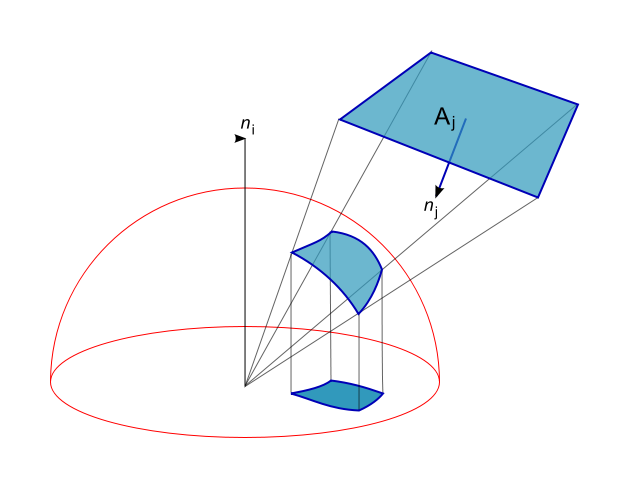Multiple Dimensions - Moving On To 3

Calculus Level 2Given that the surface area of a 3-D sphere of radius $r$ is $4 \pi r ^2$, what is the volume of the sphere?

This problem is part of Calvin's set Fun In Multiple Dimensions.

×